# SVM - Understanding the math - Part 2This is Part 2 of my series of tutorial about the math behind Support Vector Machines.
If you did not read the previous article, you might want to start the serie at the beginning by reading this article: an overview of Support Vector Machine.

In the first part, we saw what is the aim of the SVM. Its goal is to find the hyperplane which maximizes the margin.

But how do we calculate this margin?

## SVM = Support VECTOR Machine

In Support Vector Machine, there is the word vector.
That means it is important to understand vector well and how to use them.

Here a short sum-up of what we will see today:

• What is a vector?
• its norm
• its direction
• How to add and subtract vectors ?
• What is the dot product ?
• How to project a vector onto another ?

Once we have all these tools in our toolbox, we will then see:

• What is the equation of the hyperplane?
• How to compute the margin?

## What is a vector?

If we define a point $A (3,4)$ in $\mathbb{R}^2$ we can plot it like this.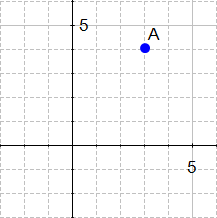Figure 1: a point

Definition: Any point $x = (x_1, x_2), x\neq0$, in $\mathbb{R}^2$ specifies a vector in the plane, namely the vector starting at the origin and ending at x.

This definition means that there exists a vector between the origin and A.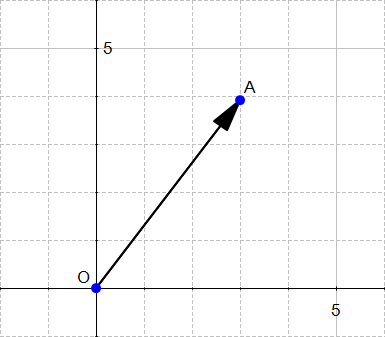Figure 2 - a vector

If we say that the point at the origin is the point $O (0,0)$ then the vector above is the vector $\vec{OA}$. We could also give it an arbitrary name such as  $\mathbf{u}$.

Note: You can notice that we write vector either with an arrow on top of them, or in bold, in the rest of this text I will use the arrow when there is two letters like $\vec{OA}$ and the bold notation otherwise.

Ok so now we know that there is a vector, but we still don't know what IS a vector.

Definition: A vector is an object that has both a magnitude and a direction.

We will now look at these two concepts.

### 1) The magnitude

The magnitude or length of a vector $x$ is written $\|x\|$  and is called its norm.
For our vector $\vec{OA}$,   $\|OA\|$ is the length of the segment $OA$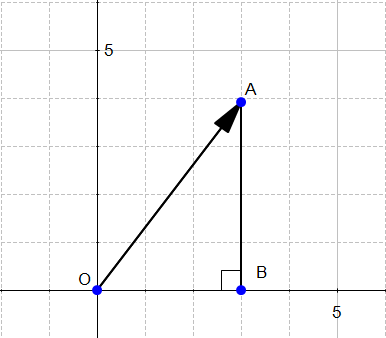Figure 3

From Figure 3 we can easily calculate the distance OA using Pythagoras' theorem:

### 2) The direction

The direction is the second component of a vector.

Definition : The direction of a vector $\mathbf{u} (u_1,u_2)$ is the vector  $\mathbf{w}(\frac{u_1}{\|u\|}, \frac{u_2}{\|u\|})$

Where does the coordinates of  $\mathbf{w}$  come from ?

#### Understanding the definition

To find the direction of a vector, we need to use its angles.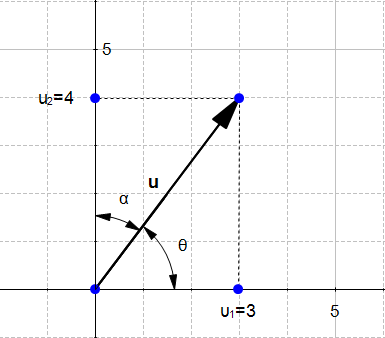Figure 4 - direction of a vector

Figure 4 displays the vector $\mathbf{u} (u_1,u_2)$ with $u_1=3$ and $u_2=4$

We could say that :

Naive definition 1: The direction of the vector $\mathbf{u}$ is defined by the angle $\theta$ with respect to the horizontal axis, and with the angle $\alpha$ with respect to the vertical axis.

This is tedious. Instead of that we will use the cosine of the angles.

In a right triangle, the cosine of an angle $\beta$ is defined by :

In Figure 4 we can see that we can form two right triangles, and in both case the adjacent side will be on one of the axis. Which means that the definition of the cosine implicitly contains the axis related to an angle. We can rephrase our naïve definition to :

Naive definition 2: The direction of the vector $\mathbf{u}$ is defined by the cosine of the angle $\theta$ and the cosine of the angle $\alpha$.

Now if we look at their values :

Hence the original definition of the vector $\mathbf{w}$ . That's why its coordinates are also called direction cosine.

#### Computing the direction vector

We will now compute the direction of the vector $\mathbf{u}$  from Figure 4.:

and

The direction of $\mathbf{u}(3,4)$ is the vector $\mathbf{w}(0.6,0.8)$

If we draw this vector we get Figure 5: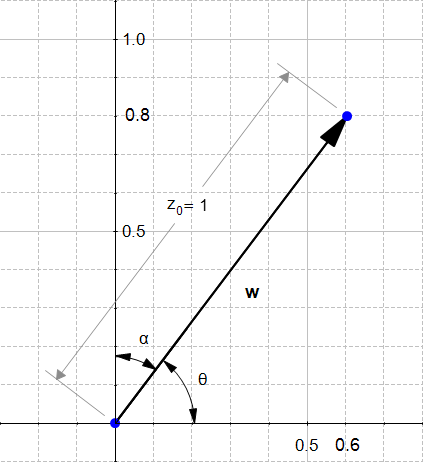Figure 5: the direction of u

We can see that $\mathbf{w}$ as indeed the same look as $\mathbf{u}$ except it is smaller. Something interesting about direction vectors like $\mathbf{w}$ is that their norm is equal to 1. That's why we often call them unit vectors.

## The sum of two vectors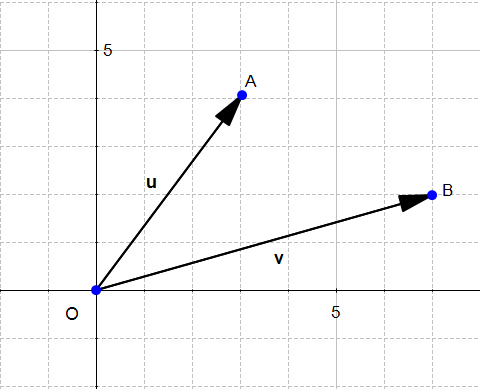Figure 6: two vectors u and v

Given two vectors $\mathbf{u} (u_1, u_2)$ and $\mathbf{v} (v_1, v_2)$ then :

Which means that adding two vectors gives us a third vector whose coordinate are the sum of the coordinates of the original vectors.

You can convince yourself with the example below:Figure 7: the sum of two vectors

## The difference between two vectors

The difference works the same way :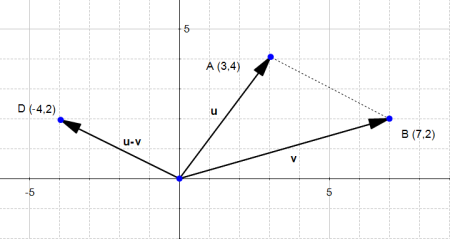Figure 8: the difference of two vectors

Since the subtraction is not commutative, we can also consider the other case: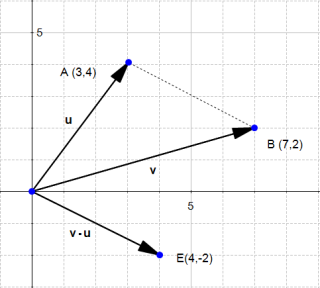Figure 9: the difference v-u

The last two pictures describe the "true" vectors generated by the difference of $\mathbf{u}$ and $\mathbf{v}$.

However, since a vector has a magnitude and a direction, we often consider that parallel translate of a given vector (vectors with the same magnitude and direction but with a different origin) are the same vector, just drawn in a different place in space.

So don't be surprised if you meet the following :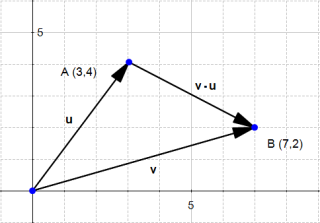Figure 10: another way to view the difference v-u

and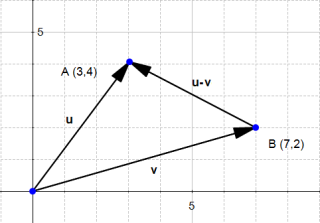Figure 11: another way to view the difference u-v

If you do the math, it looks wrong, because the end of the vector $\mathbf{u-v}$ is not in the right point, but it is a convenient way of thinking about vectors which you'll encounter often.

## The dot product

One very important notion to understand SVM is the dot product.

Definition: Geometrically, it is the product of the Euclidian magnitudes of the two vectors and the cosine of the angle between them

Which means if we have two vectors $\mathbf{x}$ and $\mathbf{y}$ and there is an angle $\theta$  (theta) between them, their dot product is :

Why ?

To understand let's look at the problem geometrically.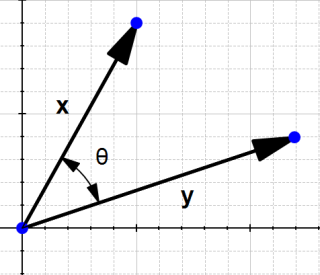Figure 12

In the definition, they talk about $cos(\theta)$, let's see what it is.

By definition we know that in a right-angled triangle:

In our example, we don't have a right-angled triangle.

However if we take a different look Figure 12 we can find two right-angled triangles formed by each vector with the horizontal axis.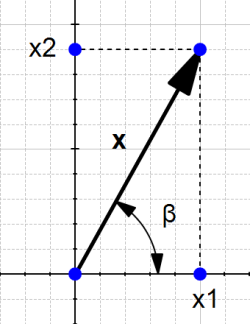Figure 13

and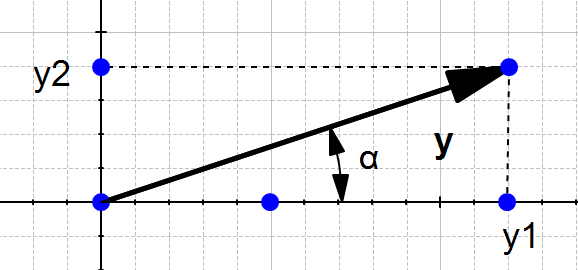Figure 14

So now we can view our original schema like this: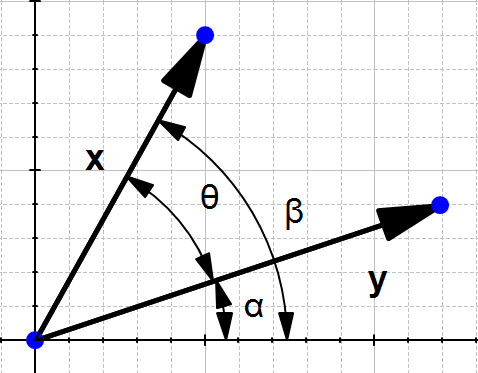Figure 15

We can see that

So computing $cos(\theta)$ is like computing $cos(\beta - \alpha)$

There is a special formula called the difference identity for cosine which says that:

(if you want you can read  the demonstration here)

Let's use this formula!

So if we replace each term

If we multiply both sides by $\|x\|\|y\|$ we get:

Which is the same as :

We just found the geometric definition of the dot product !

Eventually from the two last equations we can see that :

This is the algebraic definition of the dot product !

### A few words on notation

The dot product is called like that because we write a dot between the two vectors.
Talking about the dot product $\mathbf{x} \cdot \mathbf{y}$ is the same as talking about

• the inner product  $\langle x,y \rangle$ (in linear algebra)
• scalar product because we take the product of two vectors and it returns a scalar (a real number)

## The orthogonal projection of a vector

Given two vectors $\mathbf{x}$ and $\mathbf{y}$, we would like to find the orthogonal projection of $\mathbf{x}$ onto $\mathbf{y}$.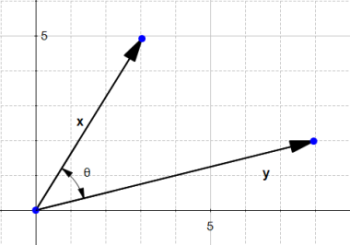Figure 16

To do this we project the vector $\mathbf{x}$ onto $\mathbf{y}$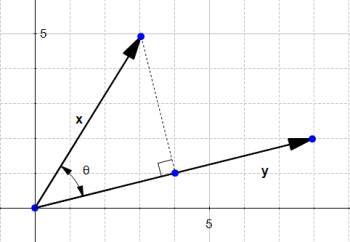Figure 17

This give us the vector $\mathbf{z}$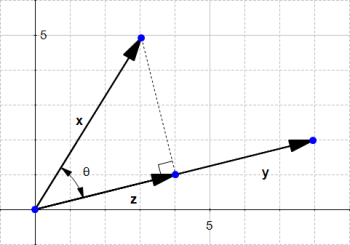Figure 18 : z is the projection of x onto y

By definition :

We saw in the section about the dot product that

So we replace $cos(\theta)$ in our equation:

If we define the vector $\mathbf{u}$ as the direction of $\mathbf{y}$ then

and

We now have a simple way to compute the norm of the vector $\mathbf{z}$.
Since this vector is in the same direction as $\mathbf{y}$ it has the direction  $\mathbf{u}$

And we can say :

The vector $\mathbf{z} = (\mathbf{u} \cdot \mathbf{x})\mathbf{u}$ is the orthogonal projection of $\mathbf{x}$ onto $\mathbf{y}$.

Why are we interested by the orthogonal projection ? Well in our example, it allows us to compute the distance between $\mathbf{x}$ and the line which goes through $\mathbf{y}$.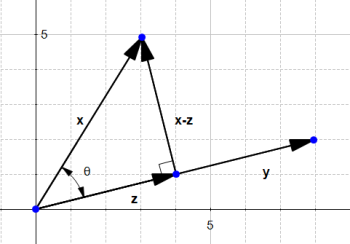Figure 19

We see that this distance is $\|x-z\|$

## The SVM hyperplane

### Understanding the equation of the hyperplane

You probably learnt that an equation of a line is : $y = ax + b$. However when reading about hyperplane, you will often find that the equation of an hyperplane is defined by :

How does these two forms relate ?
In the hyperplane equation you can see that the name of the variables are in bold. Which means that they are vectors !  Moreover, $\mathbf{w}^T\mathbf{x}$ is how we compute the inner product of two vectors, and if you recall, the inner product is just another name for the dot product !

Note that

is the same thing as

Given two vectors  $\mathbf{w}\begin{pmatrix}-b\\-a\\1\end{pmatrix}$ and $\mathbf{x}\begin{pmatrix}1\\x\\y\end{pmatrix}$

The two equations are just different ways of expressing the same thing.

It is interesting to note that $w_0$ is $-b$, which means that this value determines the intersection of the line with the vertical axis.

Why do we use the hyperplane equation $\mathbf{w}^T\mathbf{x}$ instead of  $y = ax + b$ ?

For two reasons:

• it is easier to work in more than two dimensions with this notation,
• the vector $\mathbf{w}$ will always be normal to the hyperplane(Note: I received a lot of questions about the last remark. $\mathbf{w}$ will always be normal because we use this vector to define the hyperplane, so by definition it will be normal. As you can see this page, when we define a hyperplane, we suppose that we have a vector that is orthogonal to the hyperplane)

And this last property will come in handy to compute the distance from a point to the hyperplane.

### Compute the distance from a point to the hyperplane

In Figure 20 we have an hyperplane, which separates two group of data.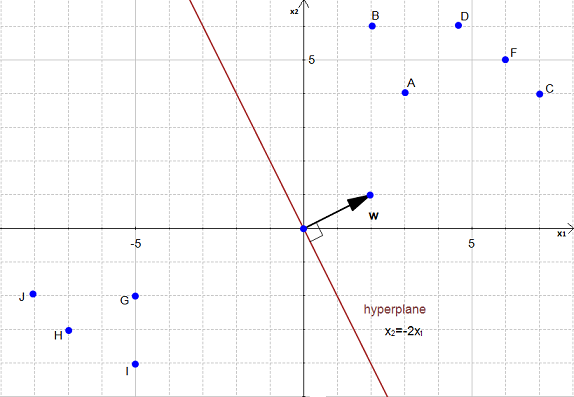Figure 20

To simplify this example, we have set $w_0 = 0$.

As you can see on the Figure 20, the equation of the hyperplane is :

which is equivalent to

with $\mathbf{w}\begin{pmatrix}2 \\1\end{pmatrix}$  and $\mathbf{x} \begin{pmatrix}x_1 \\ x_2\end{pmatrix}$

Note that the vector $\mathbf{w}$ is shown on the Figure 20. (w is not a data point)

We would like to compute the distance between the point $A(3,4)$ and the hyperplane.

This is the distance between $A$ and its projection onto the hyperplaneFigure 21

We can view the point $A$ as a vector from the origin to $A$.
If we project it onto the normal vector $\mathbf{w}$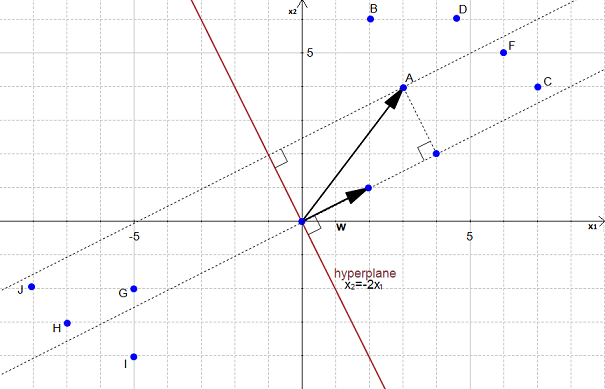Figure 22 : projection of a onto w

We get the vector $\mathbf{p}$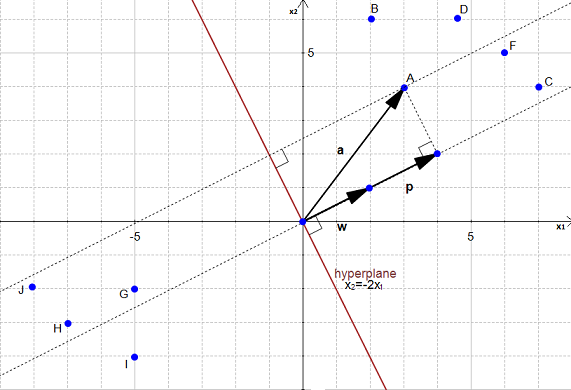Figure 23: p is the projection of a onto w

Our goal is to find the distance between the point $A(3,4)$ and the hyperplane.
We can see in Figure 23 that this distance is the same thing as $\|p\|$.
Let's compute this value.

We start with two vectors, $\mathbf{w}=(2,1)$ which is normal to the hyperplane, and $\mathbf{a} = (3,4)$ which is the vector between the origin and $A$.

Let the vector $\mathbf{u}$ be the direction of $\mathbf{w}$

$\mathbf{p}$ is the orthogonal projection of $\mathbf{a}$ onto $\mathbf{w}$ so :

## Compute the margin of the hyperplane

Now that we have the distance $\|p\|$ between $A$ and the hyperplane, the margin is defined by :

$margin = 2\|p\| = 4\sqrt{5}$

We did it ! We computed the margin of the hyperplane !

## Conclusion

This ends the Part 2 of this tutorial about the math behind SVM.
There was a lot more of math, but I hope you have been able to follow the article without problem.

### What's next?

Now that we know how to compute the margin, we might want to know how to select the best hyperplane, this is described in Part 3 of the tutorial : How to find the optimal hyperplane ?